Custom Search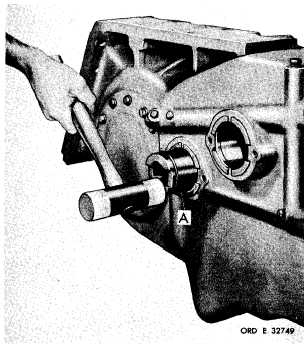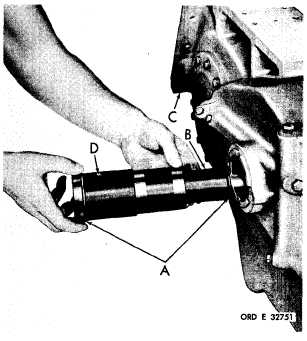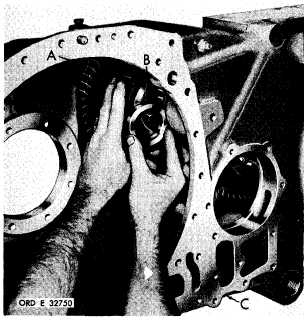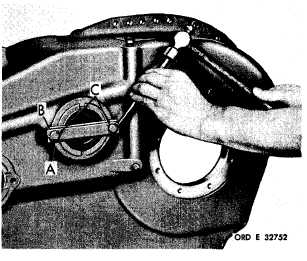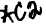*C2 C H A P    5,   S E C X X X l ll A S S E M B L Y P A R    8 ,     S T E P S    3 - 6 Figure   330   (Step   3) Install   idler   cluster   gear   spindle   assembly   (A). Figure   331   (Step   4) Install   input   transfer   cluster   gear   and   bearing a s s e m b l y    ( A )    a n d    s p a c e r    ( B ) ,    a l i n i n g    p a r ts with   the   spindle   bore   in   housing   (C). 1 8 6 Figure   332   (Step   5) I n s t a l l    O - r i n g    s e a l s    ( A )    o n t o    c l u s t e r    g e a r spindle   (B).   Install   spindle   (B)   with   seals   (A) into   housing   (C),   and   cluster   gear   and   bear- i n g    a s s e m b l y .    B e    s u r e    t h a t    o i l    h o l e    ( D )    in spindle   is   up. Figure   333   (Step   6) Install   lock   strap   (A),   lock   strip   (B)   and   two 3/8-16   x   1   bolts   (C).   Using   a   9/16-inch   wrench, t o r q u e    b o l t s    t o    2 6 - 3 2    p o u n d - f e e t .    B e n d    c o r - ners   of   strip   (B)   against   the   bolt   heads.